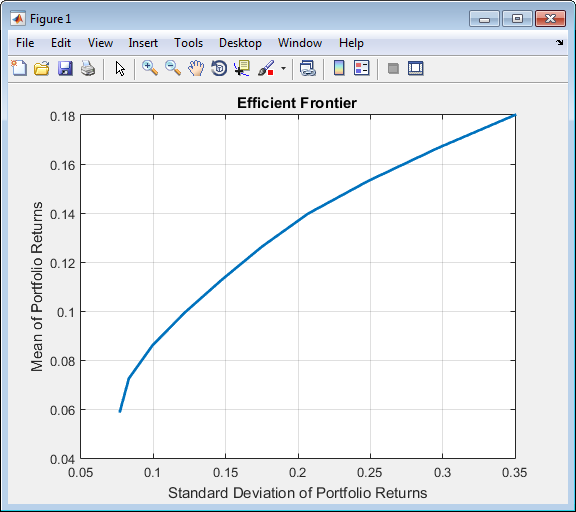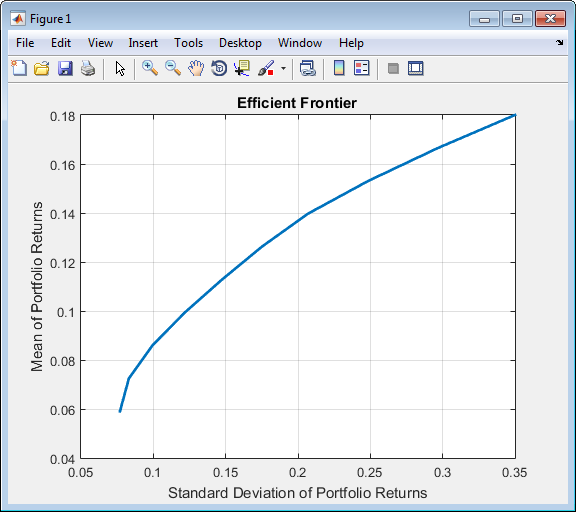## Creating the Portfolio Object

To create a fully specified mean-variance portfolio optimization problem, instantiate the `Portfolio` object using `Portfolio`. For information on the workflow when using `Portfolio` objects, see Portfolio Object Workflow.

### Syntax

Use `Portfolio` to create an instance of an object of the `Portfolio` class. You can use `Portfolio` in several ways. To set up a portfolio optimization problem in a `Portfolio` object, the simplest syntax is:

`p = Portfolio;`
This syntax creates a `Portfolio` object, `p`, such that all object properties are empty.

The `Portfolio` object also accepts collections of argument name-value pair arguments for properties and their values. The `Portfolio` object accepts inputs for public properties with the general syntax:

` p = Portfolio('property1', value1, 'property2', value2, ... );`

If a `Portfolio` object already exists, the syntax permits the first (and only the first argument) of `Portfolio` to be an existing object with subsequent argument name-value pair arguments for properties to be added or modified. For example, given an existing `Portfolio` object in `p`, the general syntax is:

`p = Portfolio(p, 'property1', value1, 'property2', value2, ... );`

Input argument names are not case-sensitive, but must be completely specified. In addition, several properties can be specified with alternative argument names (see Shortcuts for Property Names). The `Portfolio` object detects problem dimensions from the inputs and, once set, subsequent inputs can undergo various scalar or matrix expansion operations that simplify the overall process to formulate a problem. In addition, a `Portfolio` object is a value object so that, given portfolio `p`, the following code creates two objects, `p` and `q`, that are distinct:

`q = Portfolio(p, ...)`

### Portfolio Problem Sufficiency

A mean-variance portfolio optimization is completely specified with the `Portfolio` object if these two conditions are met:

• The moments of asset returns must be specified such that the property `AssetMean` contains a valid finite mean vector of asset returns and the property `AssetCovar` contains a valid symmetric positive-semidefinite matrix for the covariance of asset returns.

The first condition is satisfied by setting the properties associated with the moments of asset returns.

• The set of feasible portfolios must be a nonempty compact set, where a compact set is closed and bounded.

The second condition is satisfied by an extensive collection of properties that define different types of constraints to form a set of feasible portfolios. Since such sets must be bounded, either explicit or implicit constraints can be imposed, and several functions, such as `estimateBounds`, provide ways to ensure that your problem is properly formulated.

Although the general sufficiency conditions for mean-variance portfolio optimization go beyond these two conditions, the `Portfolio` object implemented in Financial Toolbox™ implicitly handles all these additional conditions. For more information on the Markowitz model for mean-variance portfolio optimization, see Portfolio Optimization.

### Portfolio Function Examples

If you create a `Portfolio` object, `p`, with no input arguments, you can display it using `disp`:

```p = Portfolio; disp(p); Portfolio ```
``` Portfolio with properties: BuyCost: [] SellCost: [] RiskFreeRate: [] AssetMean: [] AssetCovar: [] TrackingError: [] TrackingPort: [] Turnover: [] BuyTurnover: [] SellTurnover: [] Name: [] NumAssets: [] AssetList: [] InitPort: [] AInequality: [] bInequality: [] AEquality: [] bEquality: [] LowerBound: [] UpperBound: [] LowerBudget: [] UpperBudget: [] GroupMatrix: [] LowerGroup: [] UpperGroup: [] GroupA: [] GroupB: [] LowerRatio: [] UpperRatio: [] BoundType: [] MinNumAssets: [] MaxNumAssets: [] ```

The approaches listed provide a way to set up a portfolio optimization problem with the `Portfolio` object. The `set` functions offer additional ways to set and modify collections of properties in the `Portfolio` object.

#### Using the Portfolio Function for a Single-Step Setup

You can use the `Portfolio` object to directly set up a “standard” portfolio optimization problem, given a mean and covariance of asset returns in the variables `m` and `C`:

```m = [ 0.05; 0.1; 0.12; 0.18 ]; C = [ 0.0064 0.00408 0.00192 0; 0.00408 0.0289 0.0204 0.0119; 0.00192 0.0204 0.0576 0.0336; 0 0.0119 0.0336 0.1225 ]; p = Portfolio('assetmean', m, 'assetcovar', C, ... 'lowerbudget', 1, 'upperbudget', 1, 'lowerbound', 0);```
The `LowerBound` property value undergoes scalar expansion since `AssetMean` and `AssetCovar` provide the dimensions of the problem.

You can use dot notation with the function `plotFrontier`.

`p.plotFrontier;`#### Using the Portfolio Function with a Sequence of Steps

An alternative way to accomplish the same task of setting up a “standard” portfolio optimization problem, given a mean and covariance of asset returns in the variables `m` and `C` (which also illustrates that argument names are not case-sensitive):

```m = [ 0.05; 0.1; 0.12; 0.18 ]; C = [ 0.0064 0.00408 0.00192 0; 0.00408 0.0289 0.0204 0.0119; 0.00192 0.0204 0.0576 0.0336; 0 0.0119 0.0336 0.1225 ]; p = Portfolio; p = Portfolio(p, 'assetmean', m, 'assetcovar', C); p = Portfolio(p, 'lowerbudget', 1, 'upperbudget', 1); p = Portfolio(p, 'lowerbound', 0); plotFrontier(p);```This way works because the calls to `Portfolio` are in this particular order. In this case, the call to initialize `AssetMean` and `AssetCovar` provides the dimensions for the problem. If you were to do this step last, you would have to explicitly dimension the `LowerBound` property as follows:

```m = [ 0.05; 0.1; 0.12; 0.18 ]; C = [ 0.0064 0.00408 0.00192 0; 0.00408 0.0289 0.0204 0.0119; 0.00192 0.0204 0.0576 0.0336; 0 0.0119 0.0336 0.1225 ]; p = Portfolio; p = Portfolio(p, 'LowerBound', zeros(size(m))); p = Portfolio(p, 'LowerBudget', 1, 'UpperBudget', 1); p = Portfolio(p, 'AssetMean', m, 'AssetCovar', C); plotFrontier(p);```If you did not specify the size of `LowerBound` but, instead, input a scalar argument, the `Portfolio` object assumes that you are defining a single-asset problem and produces an error at the call to set asset moments with four assets.

#### Shortcuts for Property Names

The `Portfolio` object has shorter argument names that replace longer argument names associated with specific properties of the `Portfolio` object. For example, rather than enter `'assetcovar'`, the `Portfolio` object accepts the case-insensitive name `'covar'` to set the `AssetCovar` property in a `Portfolio` object. Every shorter argument name corresponds with a single property in the `Portfolio` object. The one exception is the alternative argument name `'budget'`, which signifies both the `LowerBudget` and `UpperBudget` properties. When `'budget'` is used, then the `LowerBudget` and `UpperBudget` properties are set to the same value to form an equality budget constraint.

Shortcuts for Property Names

Shortcut Argument Name

Equivalent Argument / Property Name

`ae`

`AEquality`

`ai`

`AInequality`

`covar`

`AssetCovar`

`assetnames` or `assets`

`AssetList`

`mean`

`AssetMean`

`be`

`bEquality`

`bi`

`bInequality`

`group`

`GroupMatrix`

`lb`

`LowerBound`

`n` or `num`

`NumAssets`

`rfr`

`RiskFreeRate`

`ub`

`UpperBound`

`budget`

`UpperBudget` and `LowerBudget`

For example, this call `Portfolio` uses these shortcuts for properties and is equivalent to the previous examples:

```m = [ 0.05; 0.1; 0.12; 0.18 ]; C = [ 0.0064 0.00408 0.00192 0; 0.00408 0.0289 0.0204 0.0119; 0.00192 0.0204 0.0576 0.0336; 0 0.0119 0.0336 0.1225 ]; p = Portfolio('mean', m, 'covar', C, 'budget', 1, 'lb', 0); plotFrontier(p); ```

#### Direct Setting of Portfolio Object Properties

Although not recommended, you can set properties directly, however no error-checking is done on your inputs:

```m = [ 0.05; 0.1; 0.12; 0.18 ]; C = [ 0.0064 0.00408 0.00192 0; 0.00408 0.0289 0.0204 0.0119; 0.00192 0.0204 0.0576 0.0336; 0 0.0119 0.0336 0.1225 ]; p = Portfolio; p.NumAssets = numel(m); p.AssetMean = m; p.AssetCovar = C; p.LowerBudget = 1; p.UpperBudget = 1; p.LowerBound = zeros(size(m)); plotFrontier(p);```